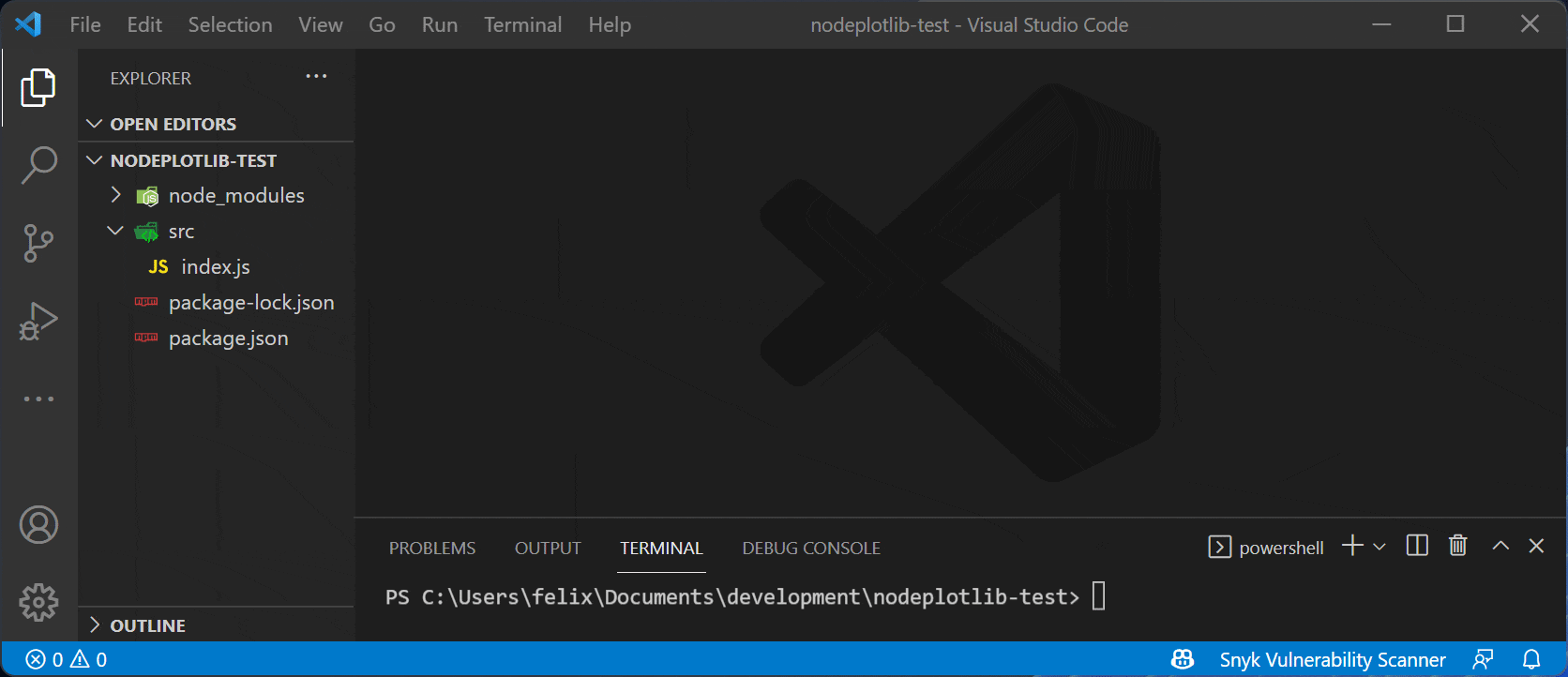#NodePlotLibLibrary to create plots directly in TypeScript or JavaScript in NodeJS on top of plotly.js without any front-end preparations. Inspired by matplotlib.

## Installation

``````npm install nodeplotlib
# or
``````

## Usage

### Creating a simple plot

Use with TypeScript/JavaScript:

``````import { plot, Plot } from "nodeplotlib";

const data: Plot[] = [
{
x: [1, 3, 4, 5],
y: [3, 12, 1, 4],
type: 'scatter',
},
];

plot(data);
``````

### Creating a stream that plots data in realtime

NodePlotLib makes use of the popular RxJS library, which provides functionality for streams, stream creator functions (e.g. from interval or from event), and tons of operators to modify your stream.

In this example we create a stream based on an interval which triggers every 100ms. Then we modify the output of the interval (which is just a counter) to be an actual `Plot` using RxJS' `map` operator. The output will be a `sin` function.

``````import { plot, Plot } from 'nodeplotlib';
import { interval, map } from 'rxjs';

const stream\$: Observable<Plot[]> = interval(100).pipe(
map(createSinusPlotFromNumber)
);

function createSinusPlotFromNumber(num: number): Plot[] {
const data: Plot[] = [
{
x: [0, 1, 2, 3, 4, 5, 6, 7, 8, 9],
y: Array(10)
.fill(0)
.map((_, i) => Math.sin(num + i)),
type: 'scatter',
},
];
return data;
}
``````

As you can see, providing a function for a dynamic plot seems to be a good idea. The functions content looks almost the same as the "non-stream" version. Simple as that, you can just put the created Observable as an argument in the plot function:

``````plot(stream\$);
``````

### API overview

There are three exports. The `plot` function and types for the Plot and for the Layout.

``````import { plot, Plot, Layout } from 'nodeplotlib';
``````

The `plot` function has the following structure

``````function plot(data: Plot[] | Observable<Plot[]>, layout?: Layout): void;
``````

It does not return a Subscription for the Observables because you just need to close the listening browser window to unsubscribe from all Obserables.

## Examples

In this section there are some examples to getting started. See the full plotly cheatsheet.

#### Line Plots

``````const trace1: Plot = { x: [1, 2], y: [1, 2], type: 'scatter' };
const trace2: Plot = { x: [3, 4], y: [9, 16], type: 'scatter' };
plot([trace1, trace2]);
``````

#### Bar Charts

``````const trace: Plot = { x: [1, 2], y: [1, 2], type: 'bar' };
plot([trace]);
``````

#### 3D Line Plots

``````const trace: Plot = {
x: [9, 8, 5, 1],
y: [1, 2, 4, 8],
z: [11, 8, 15, 3],
type: 'scatter3d',
};
plot([trace]);
``````

#### 3D Surface Plots

``````const trace: Plot = {
colorscale: 'Viridis',
z: [
[3, 5, 7, 9],
[21, 13, 8, 5],
],
};
plot([trace]);
``````

In order to style the plot, one is able to pass in the `layout` parameter, which internally is typeof `Partial<Layout>` from plotly's `Layout`. See the full layout documentation here.

With this parameter one is able to define styles like title, axis labels, subplots and many more.

``````const data: Plot[] = [
{
type: 'scatterpolar',
r: [1.5, 10, 39, 31, 15, 1.5],
theta: ['A', 'B', 'C', 'D', 'E', 'A'],
fill: 'toself',
name: 'Group B',
},
];

const layout: Layout = {
polar: {
visible: true,
range: [0, 50],
},
},
};

plot(data, layout);
``````

## Plot types

Simple charts Advanced charts 3D Plots
Scatter 2d density plots Scatter
Line Histograms Surface
Bar Box-plots Lines
Pie charts Contour plots
Sankey diagrams Heatmaps

## Contributing

Contributions in all forms are welcome.

## Developers guide

You can find the developers guide in the repositories root README.md.

## Contributors

Felix Lemke
Make. Learn. Share.
General